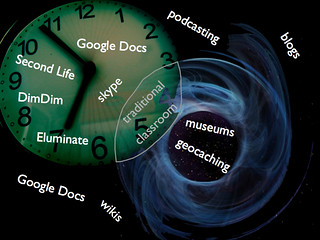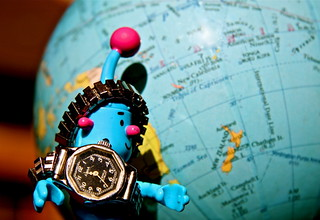# The beginning of things and the ending of things.The Big Bang era of the universe, presented as a manifold in two dimensions (1-space and time); the shape is right (approximately), but it’s not to scale. (Photo credit: Wikipedia)

The origin of the Universe, the start of everything, including time. That’s a grand concept. In our usual view of the Universe, we define any event by three space dimensions and one time dimension, but mathematics can deal with any number of dimensions. Some physical models of the Universe use many more than four dimensions, sometimes 10 or 11, and even an infinite number of dimensions.

Normally the space dimensions are depicted as being measured along three orthogonal axes, otherwise known as a Cartesian coordinate system. However there are alternate ways of specifying three space dimensions.

For instance, on the Earth’s we specify locations by latitude and longitude, which gives us two dimensions. Astronomical objects are specified by Right Ascension and Declination, again resulting in two dimensions. In both cases the third space dimension can be specified as distance, in the first case from the centre of the earth, and in the second case from the observer, but in the general case, the origin can be any arbitrary point.

This second method of specifying the position of an object is known as a Polar coordinate system, and there are many other ways that the position of an object can be specified. Of course, these positioning schemes only really work locally. If the origin of the coordinate system were on Mars for example then the coordinates of, say Jupiter, would differ from the coordinates of Jupiter as measured on Earth.

If we add time into the picture, we have four dimension to cater for. Since we live in a Universe where there appear to be three space dimensions we have difficulty in considering time to be a dimension like the three space dimensions.

A four dimensional Cartesian coordinate system seems to us to be impossible to conceive, but mathematicians don’t have any problems with the concept, and some have expended time and effort to be able to mentally conceive of four dimensional spaces.Cartesian Perspective (Photo credit: Jan Tik)

I wish them luck but I don’t see that it is necessary. The equations have four variable, hence four dimensions, end of story. There is no need to imagine four Cartesian dimensions.

Some people might consider time to be different in nature to space. It is after all measured in seconds, and space is measured in millimetres. That’s a valid point, but consider that in a Polar coordinate system the distance dimension is different to the other two dimensions. The distance is measured in millimetres and the other two in degrees or radians.Time and Space v2 (Photo credit: dkuropatwa)

It seems that it all depends on your point of view, and indeed we can measure distances in seconds too. All that we have to do is to say that the distance coordinate of an object is the number of seconds that it takes light to travel that distance. Essentially to convert from millimetres to seconds we divide by the speed of light, which, as we all learned in school is a constant.

So we have four or more dimensions in our Universe and all events in the Universe can be plotted in a space of four or more dimensions. I don’t think that it follows that every point in that space represent an event in our Universe – there may exist points in that space which don’t represent points in the space-time history of our Universe.

Consider for example a space with dimension two, which corresponds to the points on the surface of the earth (ignoring the altitude variation which corresponds the distance of the point on the surface of the earth from the centre of the earth). Every point on the surface of the earth could be plotted on Cartesian axes.

It would be a weird map with the origin representing the point on the earth which is on the equator and due South of Greenwich, the opposite side of the earth appearing at both -180 degrees and +180 degrees and the poles spread into a line at +90 degrees and -90 degrees.TTT #2… 256365 (Photo credit: paloetic)

However, no point on the earth’s surface would have a longitude coordinate that is greater than +180 or a latitude coordinate that is greater than +90. Those points just don’t represent a point on the earth’s surface. OK, the map could repeat, I guess, an infinite number of times, but I’m arbitrarily going to rule out that suggestion, as each point would not have a unique pair of coordinates.

This issue only arises because I am suggesting a mapping of Polar coordinates onto a Cartesian grid. Now I’m going to consider the four main dimensions of our Universe in a similar light.

As time is traced backwards, according to the Big Bang theory, the Universe is seen to be smaller. The further back we go, the smaller it is, until at some point the past, the whole Universe shrinks to a point. Some questioners of this theory ask “What happened before that point in time?”.

OK, let’s for the moment ignore two of the space dimensions. Let’s just consider time and, let’s say, a dimension that I’ll call width, as in the width of the Universe. We can then plot the changing width of the Universe as the vertical axis and time as the horizontal axis on a set of Cartesian axes.width & depth [CIMG2033] (Photo credit: TheAlieness GiselaGiardino²³)Arbitrarily setting the origin at now, and the width as the current width of the Universe, let’s consider what happens as we trace the graph to the left. The width, shown on the vertical axis reduces until it eventually reaches the time axis and, pop, the Universe disappears. Or appears, if we trace from left to right in the normally accepted direction of the flow of time.

“What happened one second before that point?” asks the sceptic. The answer is that the question doesn’t make sense. In the analogy above, of the latitude/longitude map, it is like asking “What is 181 degrees West of Greenwich?”, to which the answer is “There is no 181 degrees West of Greenwich.” Similarly there is no Big Bang – 1 second.How far does Hubble see? (Photo credit: NASA Goddard Photo and Video)

You will note that in that latitude/longitude example above, the map can be said to “wrap around” and if one sails 181 degrees West of Greenwich, one finds oneself at 179 degrees East of Greenwich. A similar wrap around in the case of the Universe would be for someone who somehow managed to get to Big Bang – 1 second to find that they had arrived at one second before the end of time.

However I do not know if the theories of the Big Bang allow for this possibility. Certainly the concept makes a sort of sense if the Universe is destined to collapse into a “Big Crunch”, like a Big Bang in reverse, but if the Universe is destined to expand without limit, then there is no analogy to “181 degrees West of Greenwich”.:From Image:Big_crunch.png According to the Big Crunch theory, the universe will end in an infinitely dense singularity. (Photo credit: Wikipedia)

In any case the problem likely only arises because the Cartesian coordinate system in inadequate for plotting the origin of the Universe.The Restaurant at the End of the Universe. Almost. (Photo credit: Geir Halvorsen)